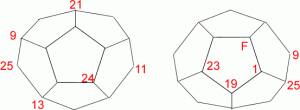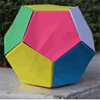Here you see the front and back views of a dodecahedron which is a solid with pentagonal faces.Using twenty of the numbers from 1 to 25, each vertex can be numbered so that the numbers around each pentagonal face add up to 65.The number F is the number of faces of the solid.
You might like to draw the net of a dodecahedron, or download the net here, and write the numbers at the vertices, or make the solid and write the numbers on it. Can you find all the missing numbers?